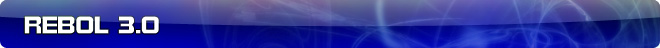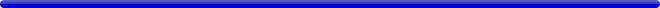Comments on: Numeric almost-equal, equal, strict-equal, and... ?

 Carl Sassenrath, CTO REBOL Technologies 22-Jun-2009 21:59 GMT Article #0211

We need to have a little discussion about numeric equality. This could get really long, so... let me boil it down.

We have these comparison functions for numbers:

• almost equal (semantically equal)
• exactly equal (bits are the same)
• strictly equal (same datatype too)

And, we have these operators (ignoring the inverted forms):

• same?
• equal?
• strictly-equal?

Now, we have to match these up, if possible. (This is a hint that perhaps we don't have all necessary operators, but let's see...)

What we need is a table that we can look at, analyze, discuss, and then agree on as the R3 standard.

On the surface, this may seem simple... but I think not. There are three dimensions. (Remember this applies across several numeric datatypes.)

To help get you thinking about it, we could begin with:

>> 0.0 = -0.0
== true
>> 0.0 == -0.0
== true
>> same? 0.0 -0.0
== false

And, then we have:

>> 0 = 0.0
== true
>> 0 == 0.0
== false
>> same? 0 0.0
== true

So... it begins.

Can you imagine the variations? We need a volunteer!